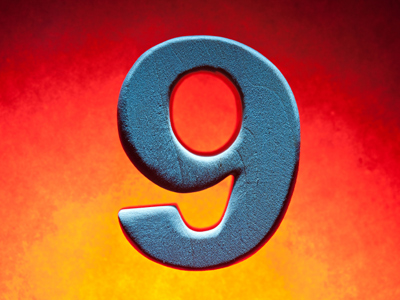The 9 times table is all about multiplying numbers by 9.

# 9 Times Table

Learning the times tables is a vital tool when multiplying numbers. By the end of Year 2 children are often fluent in their times tables up to 10. When they enter into KS2 in Year 3 they will also be taught some higher times tables in their Maths and Numeracy lessons. By the end of KS2 they will be familiar with all of the times tables from one to twelve. This quiz will test their knowledge of the 9 times table.

Learning the 9 times table involves multiplying numbers by 9. Did you know there is an easy way of multiplying by 9? Using the example of 7 x 9, take the 7 and subtract 1, making 6. That is the first of your numbers. The second is whatever is left to make 9. 6 + 3 = 9, therefore your second number is 3. So 7 x 9 = 63.

Have you been learning your times tables? Play this quiz to see how well you know your 9 times table.

1.
6 x 9 =
45
52
54
57
5 x 9 = 45 and 45 + 9 = 54
2.
11 x 9 =
90
96
99
119
To multiply numbers less than 10 by 11 just use the same number twice, so 2 x 11 = 22, 5 x 11 = 55 and 9 x 11 = 99
3.
4 x 9 =
36
38
42
43
4.
12 x 9 =
91
101
108
129
5.
9 x 9 =
18
81
83
85
A number multiplied by itself is called a square number, so 81 is 9 squared
6.
3 x 9 =
21
25
26
27
7.
5 x 9 =
42
43
45
48
To multiply a number by 5 first times it by 10 and then half it, so 9 x 10 = 90 and 90 ÷ 2 = 45
8.
2 x 9 =
11
18
20
22
To multiply a number by 2 just double it
9.
8 x 9 =
72
73
76
78
10.
7 x 9 =
60
61
63
69
You can find more about this topic by visiting BBC Bitesize - 9 times table and division facts

Author:  Amanda Swift